## The gravitational force law, deduced by Newton in the 1660’s, is remarkably similar to Coulomb’s law. Recall that the universal law of gravi

Question

The gravitational force law, deduced by Newton in the 1660’s, is remarkably similar to Coulomb’s law. Recall that the universal law of gravitation states that the magnitude of the gravitational force between two masses M1 and M2 separated by a distance R is given by the following equation:________.
F = G (M1 x M2) / R2
G = 6.67 x 10-11 Nm2/kg2
a. Calculate the value of the gravitational force between an electron (mass = 9.11 x 10-31 kg) and a proton (mass is 1836 times greater than the mass of an electron) if the two particles are separated by 3.602 nanometers. (1 nanometer or 1 nm = 1 x 10-9 m)
F= ______ N
b. The force created in the above question is:
1. repulsive
2. attractive

in progress 0
6 months 2021-08-25T19:33:21+00:00 1 Answers 3 views 0

## Answers ( )

a.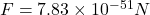b.Attractive

Explanation:

We are given that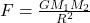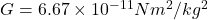Mass of an electron,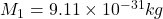Mass of proton,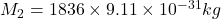Distance between electron and proton,R=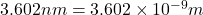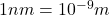a.Substitute the values then  we get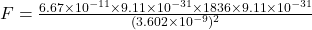b.We know that like charges repel to each other and unlike charges attract to each other.

Proton and electron are unlike charges therefore, the force between proton and electron is attractive.